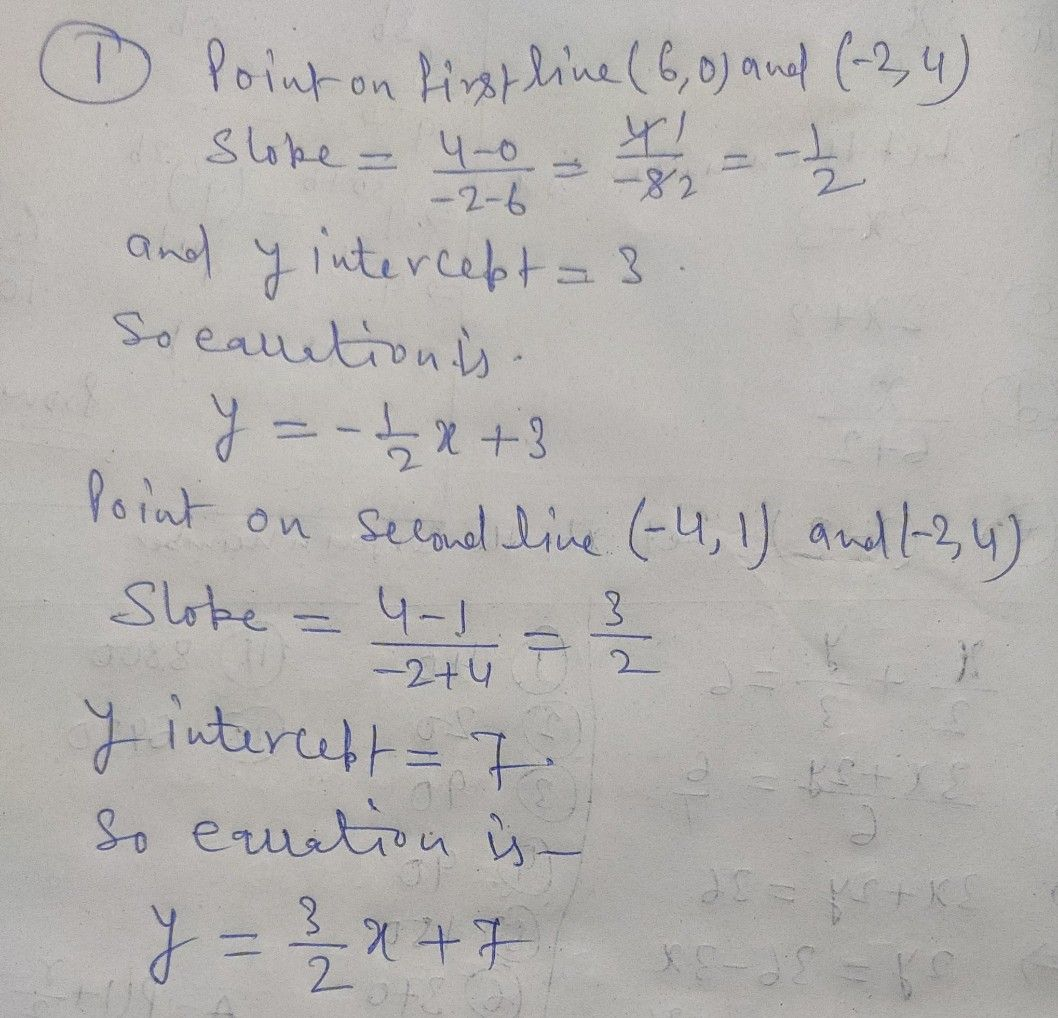Symbol
Problem$3$ arning Task $4:D0$ $i1$ in your notebook. Describe the $s1$ slope and $y-$ intercepts of the graphs of the system of equations. $2.$
10th-13th grade
Other
Search count: 105
SolutionQanda teacher - Ranjeetif you have no query regarding this question, please evaluate the answer with your reviewStudent
how about of number two sir? where is the solutio?Qanda teacher - Ranjeet
As like 1 you can do it. Take any two point on each line and do as 1Student
ok sir thank very muchQanda teacher - Ranjeet
please evaluate the answer with your review